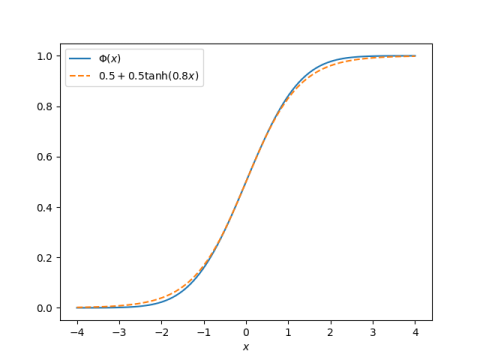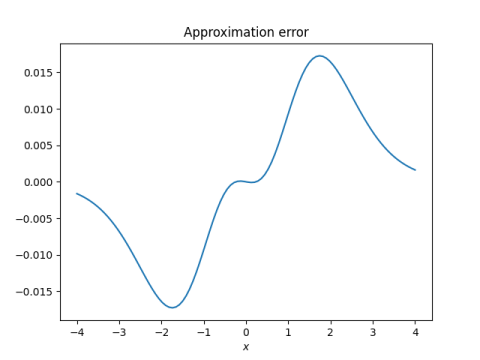# Computing normal probabilities with a simple calculator

If you need to calculate Φ(x), the CDF of a standard normal random variable, but don’t have Φ on your calculator, you can use the approximation 

Φ(x) ≈ 0.5 + 0.5*tanh(0.8 x).

If you don’t have a tanh function on your calculator, you can use

tanh(0.8x) = (exp(1.6x) – 1) / (exp(1.6x) + 1).

This saves a few keystrokes over computing tanh directly from its definition .

## Example

For example, suppose we want to compute Φ(0.42) using `bc`, the basic calculator on Unix systems. `bc` only comes with a few math functions, but it has a function `e` for exponential. Our calculation would look something like this.

```    > bc -lq
t = e(1.6*0.42); (1 + (t-1)/(t+1))/2
.66195084790657784948
```

The exact value of Φ(0.42) is 0.66275….

## Plots

It’s hard to see the difference between Φ(x) and the approximation above.A plot of the approximation error shows that the error is greatest in [-2, -1] and [1, 2], but the error is especially small for x near 0 and x far from 0.## Related posts

 Anthony C. Robin. A Quick Approximation to the Normal Integral. The Mathematical Gazette, Vol. 81, No. 490 (Mar., 1997), pp. 95-96

 Proof: tanh(x) is defined to be (exex) / (ex + ex). Multiply top and bottom by ex to get (e2x – 1) / (e2x + 1).